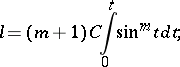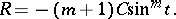# Ribaucour curve

(diff) ← Older revision | Latest revision (diff) | Newer revision → (diff)

A planar curve with curvature radiusat an arbitrary pointproportional to the length of the segment of the normal MP (see Fig.).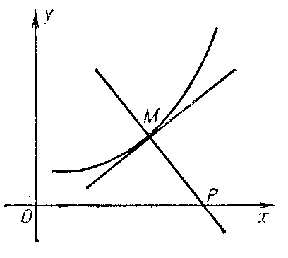Figure: r081760a

The equation for the Ribaucour curve in Cartesian orthogonal coordinates iswhere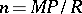. If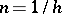(is any integer), then a parametric equation for the Ribaucour curve is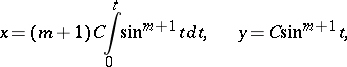where. When, the Ribaucour curve is a circle; when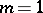, it is a cycloid; when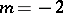, it is a catenary; and when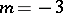, it is a parabola.

The length of an arc of the curve is